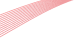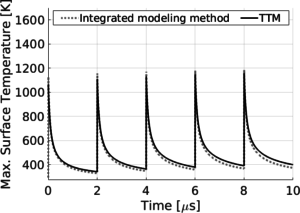# Integrating two-temperature and classical heat accumulation models to predict femtosecond laser processing of silicon

Lauren L. Taylor, Ryan E. Scott, and Jie QiaoA robust, computationally efficient modeling method describing multi-pulse femtosecond laser-material interaction is required to determine the optimal laser parameters to control and differentiate non-thermal ablation and heat accumulation processes for surface structuring and laser welding applications. We establish a three-dimensional, two-temperature model (TTM) and a heat-accumulation model based on classical heat generation and conduction equations to evaluate their efficacy and efficiency in simulating non-thermal ablation and heat accumulation during multi-pulse femtosecond laser processing of silicon. Only the TTM is capable of accurately predicting the laser fluences required to achieve non-thermal ablation, which is experimentally validated. Both the TTM and the classical heat accumulation model can predict heat accumulation. The TTM can accurately predict heat accumulation, but requires lengthy simulation times on the order of several hours. The classical heat accumulation model consistently predicts heat accumulation with the TTM and is time efficient, but is case specific to interaction parameters, requiring input of an experimentally-determined absorption coefficient. For the first time to our knowledge, an integrated modeling method is devised to accurately and efficiently simulate laser-processing-induced heat accumulation by using the TTM to determine an absorption coefficient to feed back to the heat accumulation model to extend it to the general case. This integrated modeling method enables the accurate prediction of heat accumulation with simulation times on the order of a minute per pulse, defining a path to determine laser parameters to control heat accumulation for specific processing applications.